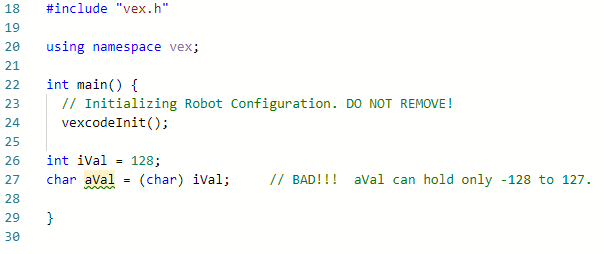# Creating Float and Double Variables in VEXcode Pro V5

## Types that hold numbers with decimal places – float, double

These data types are often called floating-point data types. The numbers are referred to as floating-point numbers.

```float fvar = 50.15;
double dvar = 50.0;
```

## Compare floating-point data type – float and double

The difference between a float and double data type is all about the precision on the # of decimal places.

Based on the VexOS – ARM 7 specification, float can handle 6 or 7 places, and double can handle 15 or 16.

float: in the current V5os, the controller seems to allow up to 8 decimal places of precision.

e.g.

```float fvar1 = 1.12345670;
float fvar2 = 1.12345678;
```

fval1 is the different from fvar2,

v.s.

```float fvar1 = 1.123456780;
float fvar2 = 1.123456781;
```

fval1 will be evaluated the same as fvar2.

double: allows up to 17 decimal places of precision.

e.g.

```double dvar1 = 1.12345678912345678;
double dvar2 = 1.12345678912345670;
```

dval1 is different from dvar2,

v.s.

```double dvar1 = 1.123456789123456789;
double dvar2 = 1.123456789123456780;
```

dval1 will be evaluated the same as dvar2.

## Do not use "double" unless you need high precision

There are two reasons you shouldn't use "double" unless you need high precision:

1. It takes up 2X float type.
2. It takes up much higher processing power.

Based on the VexOS – ARM 7 specification, a float can do 6 or 7 places, and a double can handle 15 or 16. In either case, you should not rely on the accuracy of the last digits in double or float. It is stored very differently from storing an “int” due to something called “float-point calculation” (which is outside the scope of this article).

Thus, you may possibly lose the accuracy at the last few decimal places.

Operation on double is quite costly compared to float,  and much higher than “integer” due to something called floating-point calculations.

Based on IEEE standard, the following is within a more reliable range.

 Type Smallest Positive Value Largest Positive Value # of Precision float 10 -38 x 1.17549 10 38 x 3.40282 6 digits double 10 -308 or 2.22507 10 308 x 1.79769 15 digits

## Can you interchange primitive data types? - Casting

It is possible to interchange data types. This technique is called “Casting.” While casting is a rather common practice, do that only if it is necessary.

##### One of the most common practices is to exchange char and int.

This is because they both represent integer values with one exception:

• “int” contains 4-bytes (or 8-bytes in some compiler)
• “char” contains only 1-byte.

So, e.g.

```char aVal = 100;
int iVal = aVal;   // implicit casting
```

or

```int iVal = (char) aVal;   // explicit casting.
```

Caution for implicit truncation:

e.g.##### Another common practice is to exchange int and float
```float fVal = 5.486;
float fraction =  fVal - (int)fVal;   //it does: 5.486 - 5
```

Caution for implicit truncation:

e.g.

```int iVal = 50;
float fVal = iVal / 100;
```

now fVal = 0.0, NOT 0.5.

It is absolutely fine to take advantage of implicit truncation, which is often an important part of operations in some scenarios. However, you need to make sure that you're using this technique by design.

If you do not wish implicit truncation to take place, you need to do explicit casting:

• implicit casting: `float fVal = iVal / 100.0;`
• explicit casting: `float fVal = (float) iVal / 100`

For more information, help, and tips, check out the many resources at VEX Professional Development Plus

Last Updated: